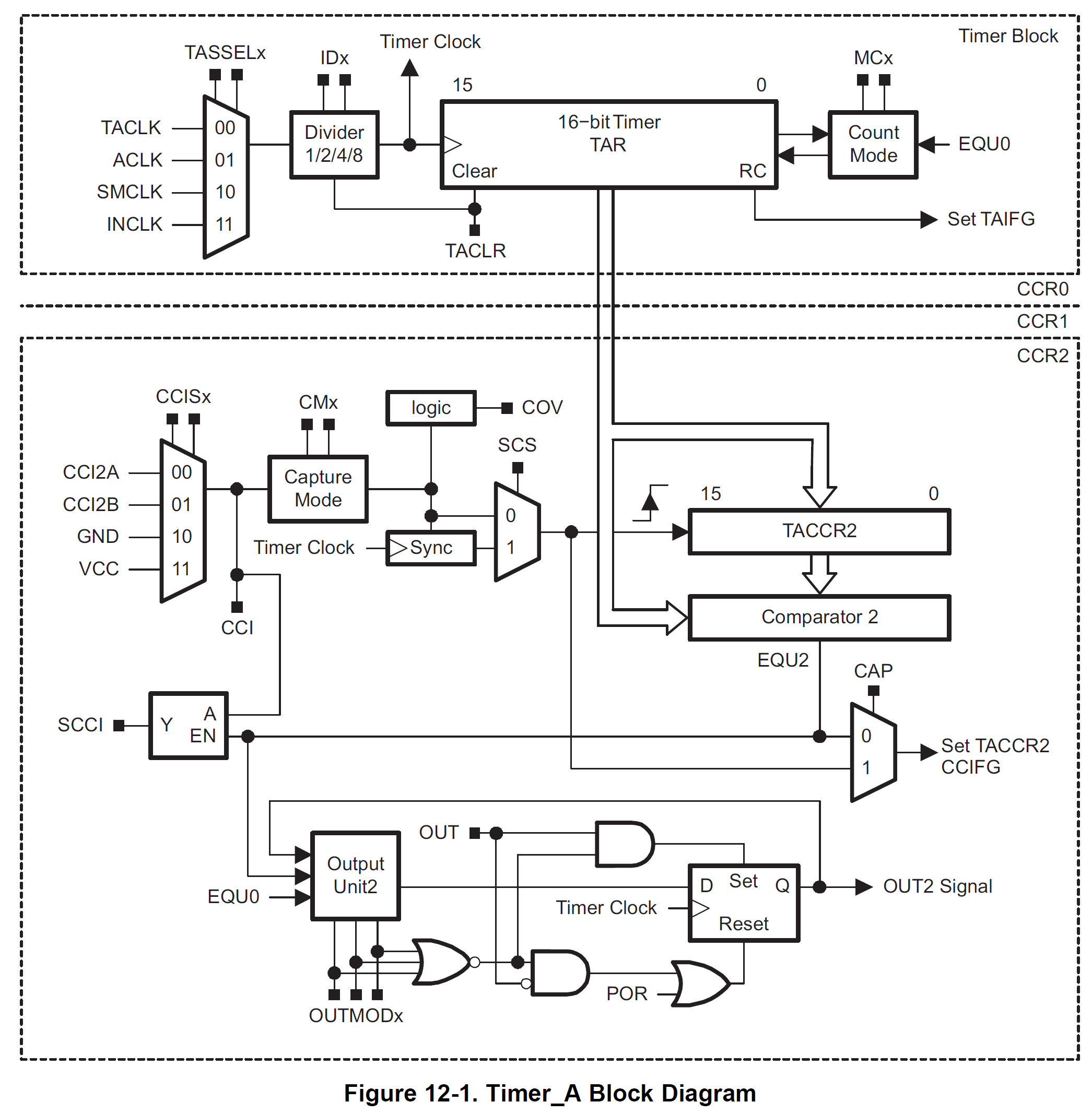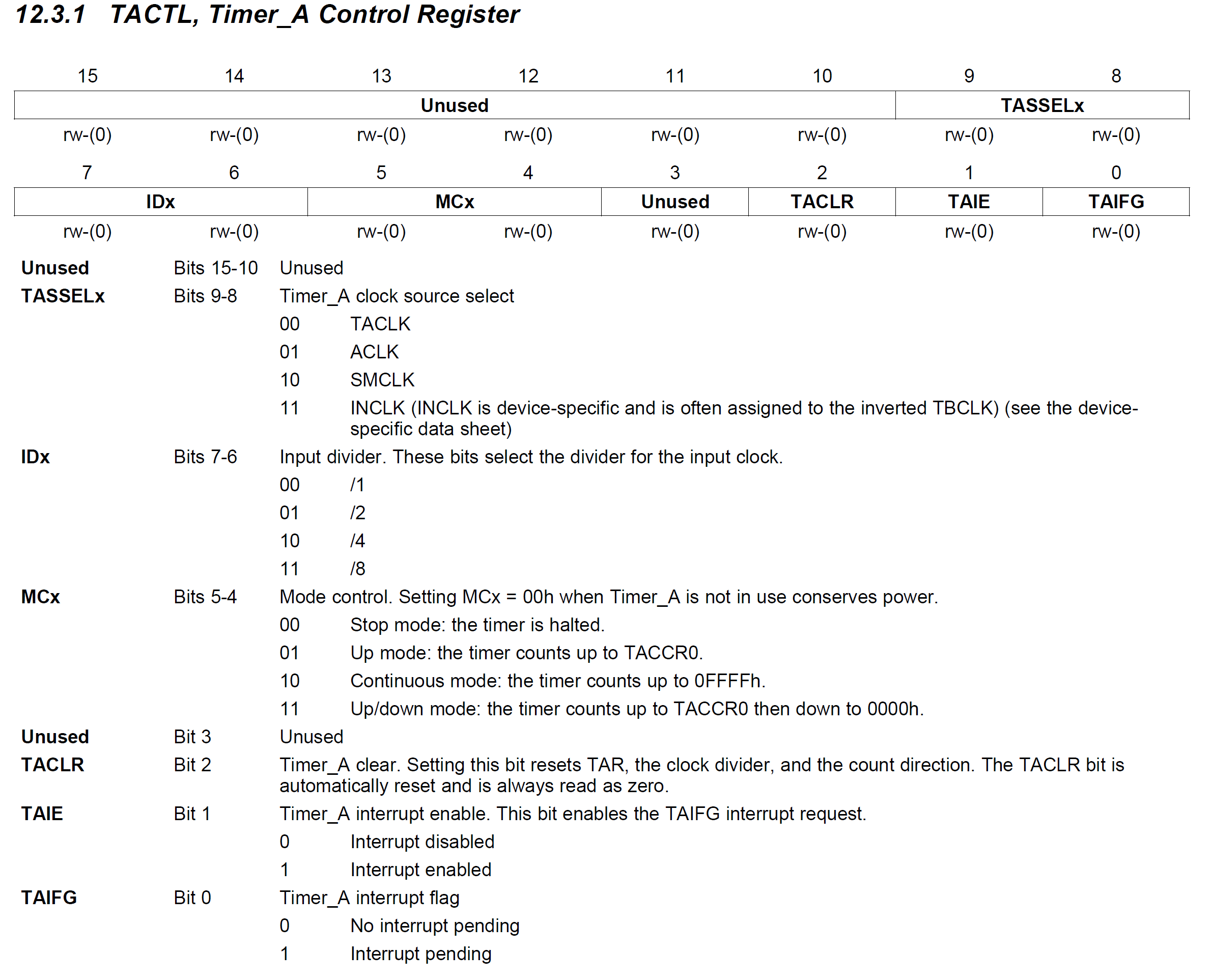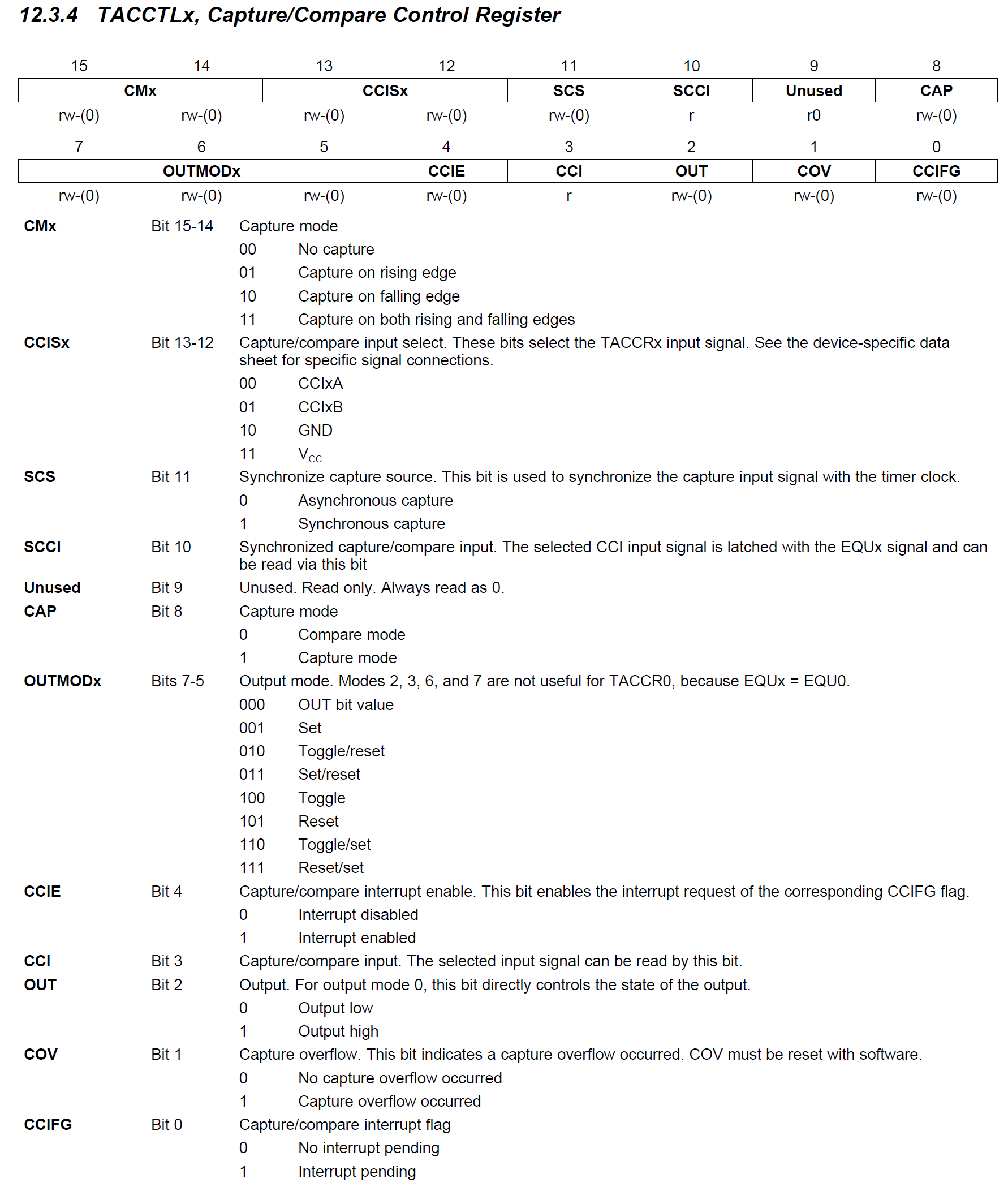# Timer A

## Overview• Multiple input clock sources: mainly ACLK or SMCLK
• 16-bit timer: Counts from 0 to 0xFFFF or 65535
• 3 so-called capture and compare register CCR0 to CCR2
• Capture mode:
• Copying timer's value to CCR on interrupt
• e.g. Measuring signal's frequency or periode
• Compare mode:
• Generating a interrupt, when timer's value is equal to CCR
• Automatically setting/toggling an output signal on equality
• Generating:
• symmetrical square waves (e.g. tone generator)
• PWM (pulse-with-modulated) signals (e.g. LED dimming, motor control, ...)
• Registers to control Timer A's functions:Info

Timer A0 and A1 of MSP430G2553

The MSP430G2553 has two Timer A. In the following table the resulting register and interrupt names can be seen.

Description Timer A0 Timer A1
Timer A Control Register TA0CTL TA1CTL
Timer A Capture&Compare Register 0 TA0CCR0 TA1CCR0
Timer A Capture&Compare Register 1 TA0CCR1 TA1CCR1
Timer A Capture&Compare Register 2 TA0CCR2 TA1CCR2
Timer A Capture&Compare Control Register 0 TA0CCTL0 TA1CCTL0
Timer A Capture&Compare Control Register 1 TA0CCTL1 TA1CCTL1
Timer A Capture&Compare Control Register 2 TA0CCTL2 TA1CCTL2
Timer A Interrupt Vector 0 TIMER0_A0_VECTOR TIMER1_A0_VECTOR
Timer A Interrupt Vector 1 TIMER0_A1_VECTOR TIMER1_A1_VECTOR

## Generating Continuous Interrupt Signals

### Using the CCR0

Generation of an interrupt signal using CCR0
int main(void) {
WDTCTL = WDTPW | WDTHOLD;  // stop watchdog timer

P1DIR |= BIT0;

TA0CCR0 = 0;
TA0CCTL0 = CCIE;
TA0CTL = TASSEL_1 + MC_2 + TACLR;

__enable_interrupt();

while (1) {
__low_power_mode_3();
}
}

#pragma vector=TIMER0_A0_VECTOR
__interrupt void TIMER0_A0_ISR() {
P1OUT ^= BIT0;
}

• Setting the bits in the registers TA0CCTL0 and TA0CTL as needed.
• Using the constants provided by the msp430.h named after the datasheet.

Info

Multi-bit naming convention in msp430.h
Single-bit constants can be directly used as named in the datasheet.
e. g. TACLR is equal to BIT2 or 0x04

Multi-bit constants need to be distinguished between underscore and no-underscore convention. The following table is showing the difference in the mode control bits.

Value Description Underscore Convention No-Underscore Convention
00 Stop Mode MC_0 0
01 Up Mode MC_1 MC0
10 Continuous Mode MC_2 MC1
11 Up/Down Mode MC_3 MC1 + MC0

Analysing the Program

• TA0CCR0 = 0;
• Setting the CCR to 0.
• Every time the timer counter will reach the value 0, a event will occur.
• TA0CCTL0 = CCIE;
• Compare mode → CAP = 0
• Enabling the Interrupt → CCIE = 0
• TA0CTL = TASSEL_1 + MC_2 + TACLR;
• use ACLK = 32.768 kHz → TASSEL = 01
• no clock divider → ID = 00
• starting timer in continuous mode → MC = 10
• clearing the timer counter → TACLR = 1
• __low_power_mode_3();
• Enter LPM 3.
• ACLK needs to remain active, see Figure 3.2.
• #pragma vector=TIMER0_A0_VECTOR
Interrupt Vector for the CCR0 interruptInfo

Calculation of Timer Delays

Formular:

$\Delta t = \frac{\Delta n}{f}$

with $$\Delta t$$ the time between two events, $$\Delta n$$ the difference of the timer counter, or CCR value and $$f$$ the input clock frequency of the timer.

With $$f = 32.768\si{kHz}$$ the resulting delay times are:

Δn (hex) Δn (dec) Δt [ms]
0x4000 16384 500
0x8000 32768 1000
0xC000 49152 1500
0xFFFF 65535 1999.97
0x10000 65536 2000

• In order to let the LED blink with an frequency of 1 Hz, the LED state needs to be changed (P1OUT ^= BIT0;) once every 500 ms.
• After the timer counted to 0x4000, an event needs to occur.
• Solution 1: Using the Up Mode of the Timer
• Up mode: the timer counts up to TACCR0
• setting TA0CCR0 to 0x4000 will generate an event every 500 ms.
Changing the blinking frequency to 1 Hz - using the Up Mode of the Timer
TA0CCR0 = 0x4000;
TA0CCTL0 = CCIE;
TA0CTL = TASSEL_1 + MC_1 + TACLR;

• Solution 2: Using the Continuous Mode of the Timer
• Leaving the Timer in the Continuous Mode is a good practice in order to use CCR1 and CCR2 the same way. This can be seen in the following subsection.
• The ideas is to constantly change the CCR value in order to capture the next event at the correct time.
• In order to get correct interrupt at first the CCR0 needs to be 0x4000.
• To get an interrupt after 500 ms the next value needs to be 0x8000, then 0xC000, 0x0000 and 0x4000 again.
• This value change can be automatically calculated by executing TA0CCR0 += 0x4000.
• The overflow from 0xC000 + 0x4000 to 0x0000 is automatically done, because TA0CCR0 is a 16bit register.Changing the blinking frequency to 1 Hz - using the Continuous Mode of the Timer
TA0CCR0 = 0x4000;
TA0CCTL0 = CCIE;
TA0CTL = TASSEL_1 + MC_2 + TACLR;

...

#pragma vector=TIMER0_A0_VECTOR
__interrupt void TIMER0_A0_ISR() {
P1OUT ^= BIT0;
TA0CCR0 += 0x4000;
}


### Using all CCR and the Timer Overflow Interrupt

• CCR1 and CCR2 can be used to generate interrupts, too.
• These interrupts are enabled by setting the CCIE flag in the corresponding TA0CCTLx register.
• Another interrupt source is the timer overflow. If the timer reaches the value 0xFFFF a interrupt is generated.
• This interrupt is enabled by setting the TAIE flag in the TA0CTL register.
• The following test program lets the RGB LEDs blink at different rates. Because the frequency is doubled from LED to LED all possible RGB colors will appear.
• The green LED (P1.0) will be toggled on timer overflow - once every two seconds.
Using all CCR and the Timer Overflow Interrupt
int main(void) {
...

P1OUT &= ~BIT0;
P2OUT &= ~(BIT1 + BIT3 + BIT5);
P1DIR |= BIT0;
P2DIR |= BIT1 + BIT3 + BIT5;

TA0CCR0 = 0x2000;
TA0CCTL0 = CCIE;
TA0CCR1 = 0x4000;
TA0CCTL1 = CCIE;
TA0CCR2 = 0x8000;
TA0CCTL2 = CCIE;
TA0CTL = TASSEL_1 + MC_2 + TACLR + TAIE;

...
}

#pragma vector=TIMER0_A0_VECTOR
__interrupt void TIMER0_A0_ISR() {
P2OUT ^= BIT1;
TA0CCR0 += 0x2000;
}

#pragma vector=TIMER0_A1_VECTOR
__interrupt void TimerA1_ISR() {
switch (TAIV) {
case 2:
// CCR1 Interrupt
P2OUT ^= BIT3;
TA0CCR1 += 0x4000;
break;
case 4:
// CCR2 Interrupt
P2OUT ^= BIT5;
TA0CCR2 += 0x8000;
break;
case 10:
// Timer Overflow
P1OUT ^= BIT0;
break;
}
}

• Because the timer can generate four different interrupts (3 by CCR + timer overflow) it can be expected that there are four different interrupt vectors and ISR.
• But the MSP430 only uses two ISR per timer to safe adress space.
• As you know from the port interrupts, ISR can be used by different interrupts sources. The interrupts flags needs to be read in order to identify the interrupts source.
• This is also true for the seconds ISR of the timer: vector=TIMER0_A1_VECTOR
• This ISR is used by the CCR1, CCR2 and timer overflow interrupt.
• The following switch case structure should be used to identify the interrupt source:

switch (TAIV) {
case 2:
// CCR1 Interrupt
break;
case 4:
// CCR2 Interrupt
break;
case 10:
// Timer Overflow
break;
}Question

Modify the program using all CCRs to use only the Timer A1 instead of Timer A0. Change the corresponding register and interrupt vector names.